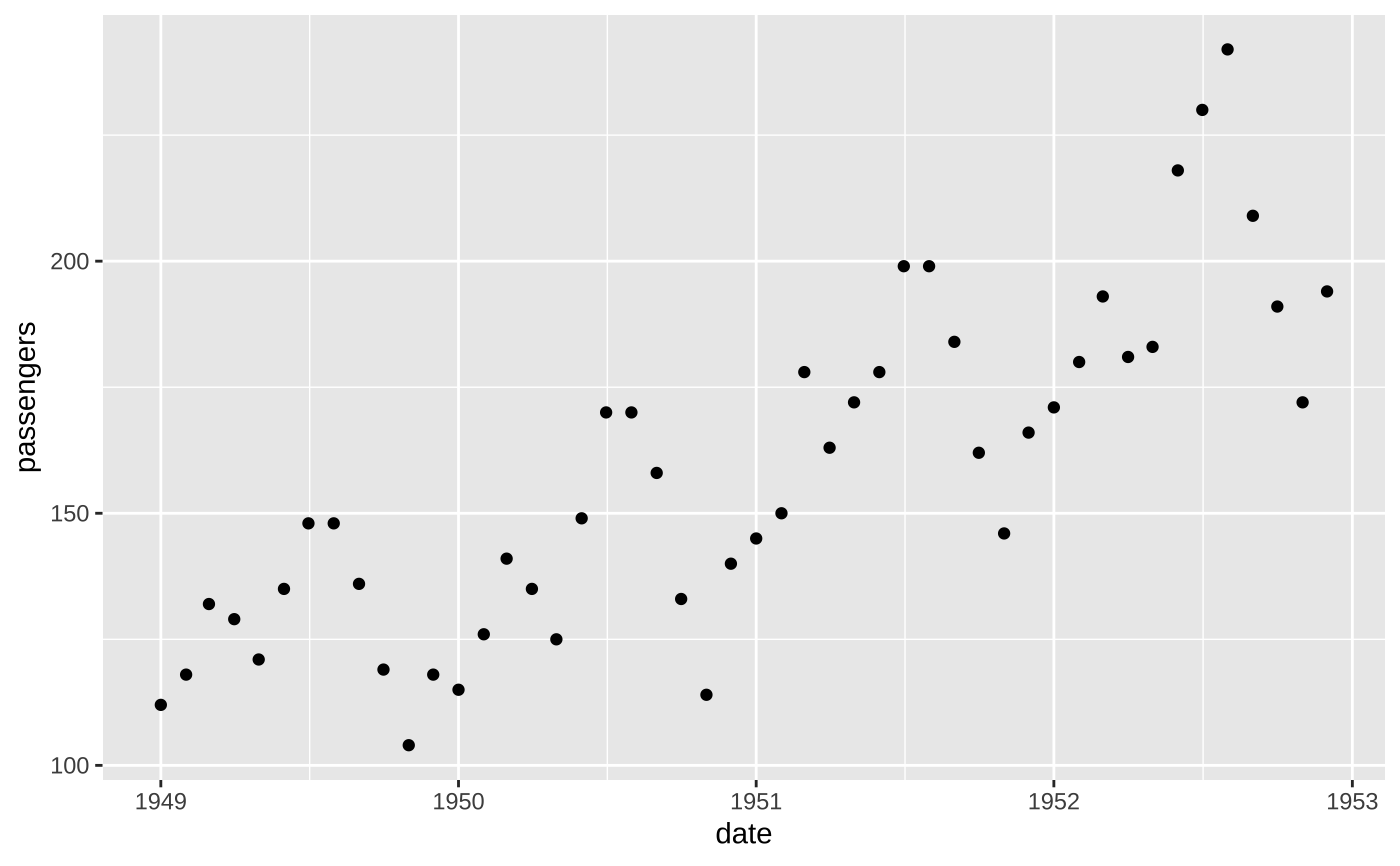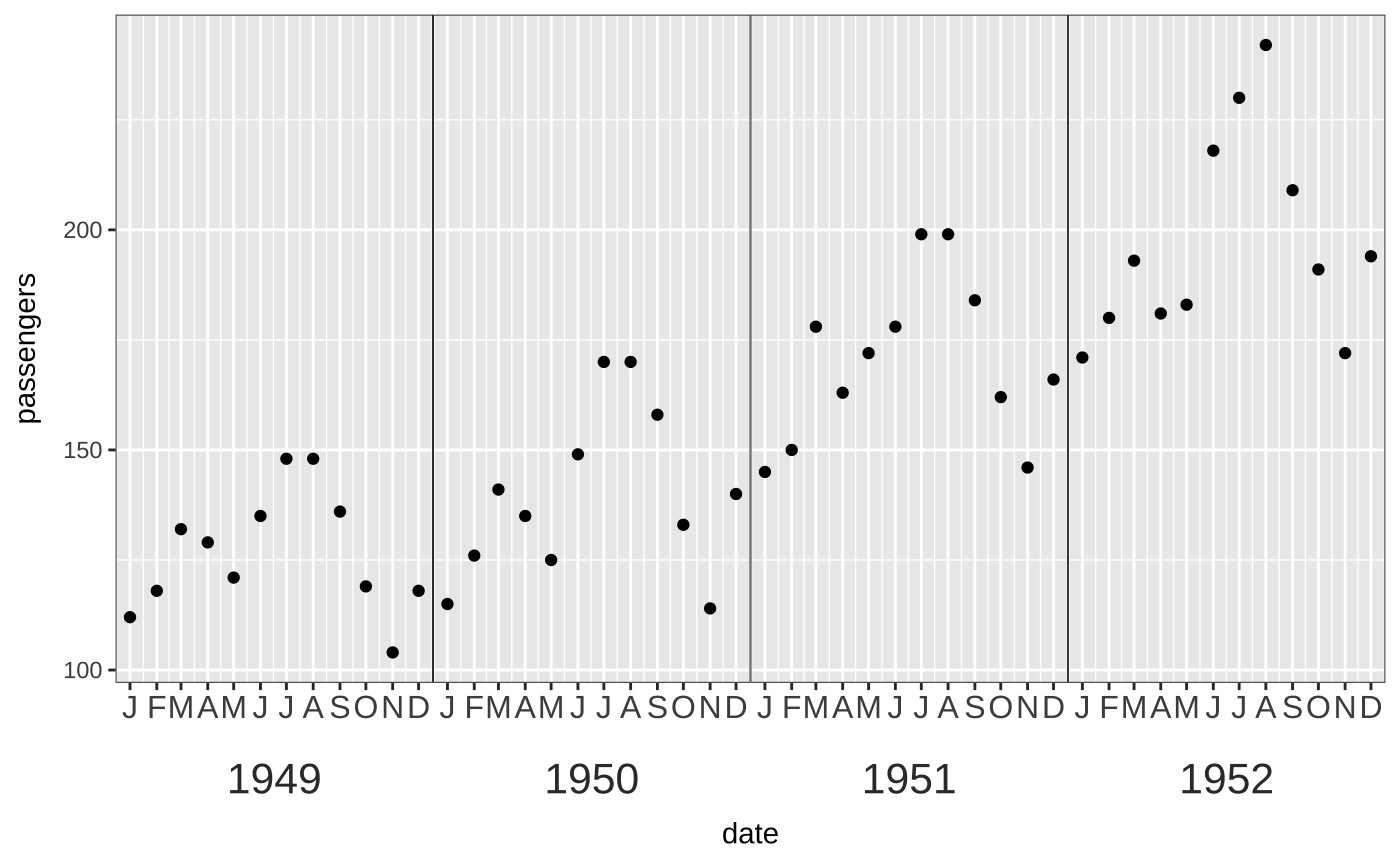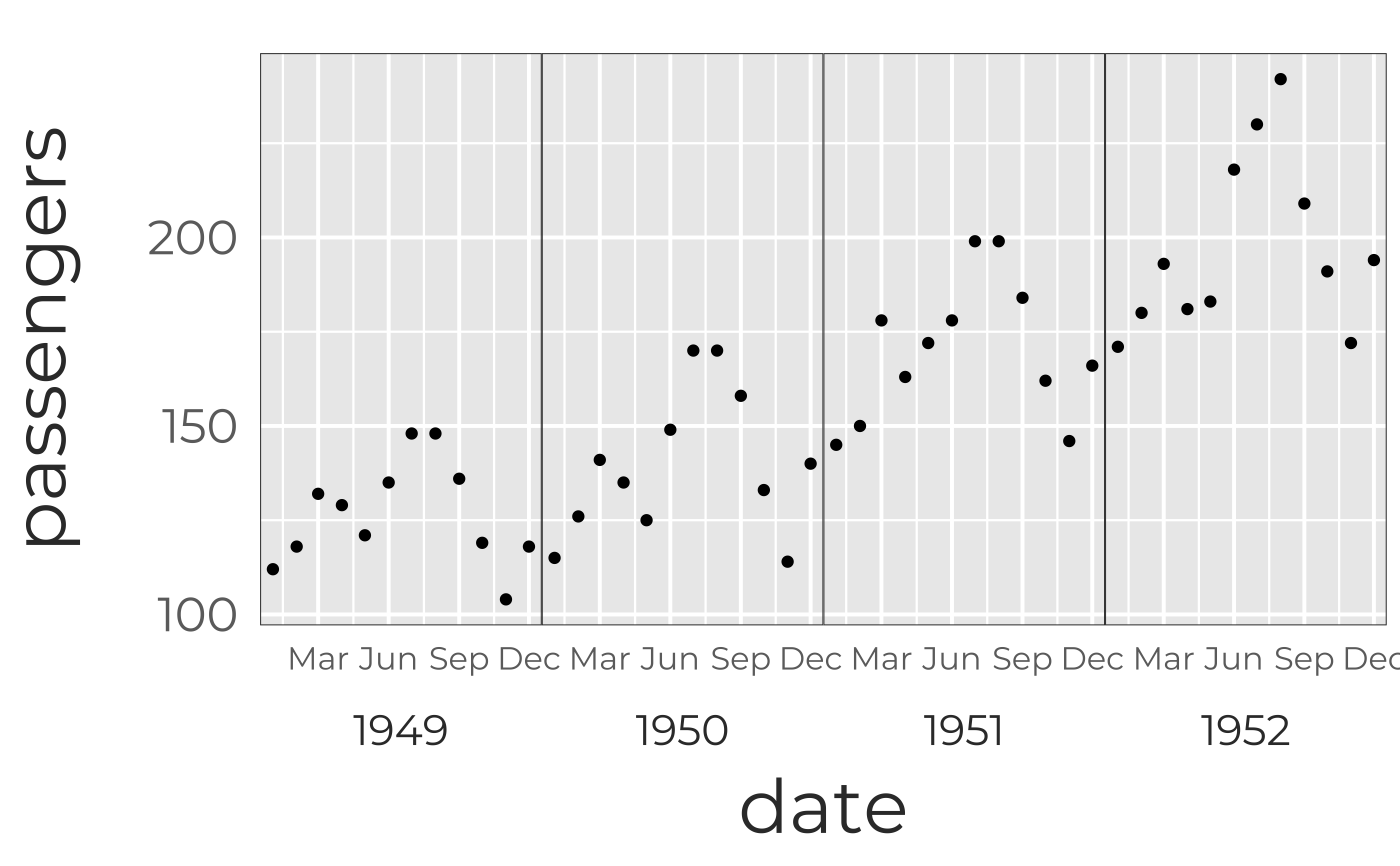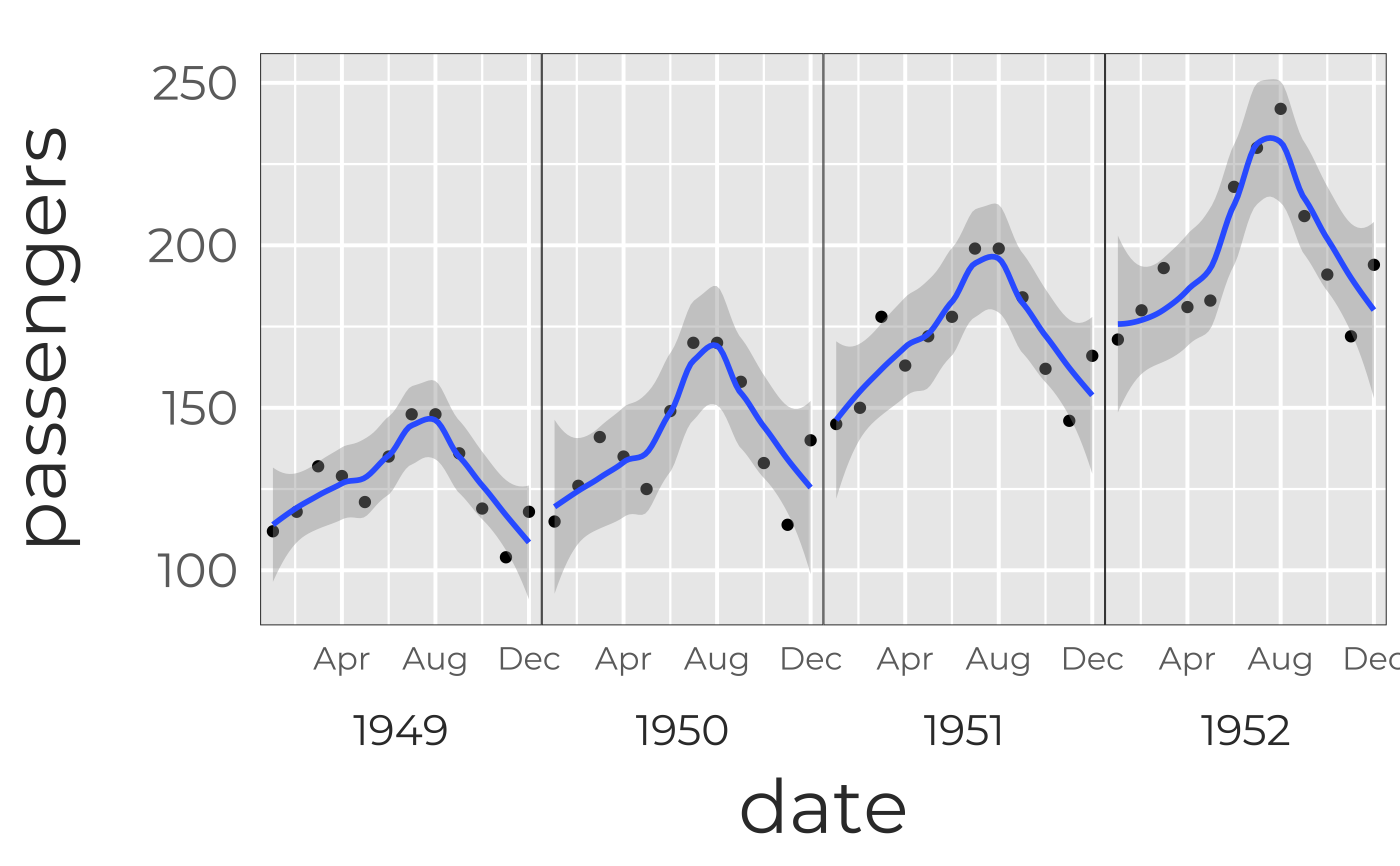Makes y-axis have year as a bottom facet, with abbreviated months as minor axis-labels.

scale_x_yearmonth(
abbrev = 1,
breaks.mnth = 1,
mnth.size = 12,
yr.var = "Year",
yr.size = 16,
...
)

## Arguments

abbrev How long to make months? Default=1 means just first letter of month; NA is full month name. Frequency of month labels; default= 1 (every month) Font size of month labels; default=12 (pts) Variable name for year; default= "Year" Font size of year labels; default=16 (pts) other arguments for scale_x_date

## Details

Code inspired by This post by Jason Fabris

## Examples

require(ggplot2)
#Set up time series dataset
df<-data.frame(date=as.Date(c(sapply(1949:1952,function(yr) {paste(yr,1:12,1,sep="-")}))),
passengers=as.vector(AirPassengers)[1:48])
df$year<-sprintf("%.4s",df$date)
(g <- ggplot(df,aes(date,passengers))+geom_point())#Now break show months and year on x-axis
#(doesn't work, because it expects a year variable called "Year")
if (FALSE) {
(g2 <- g+scale_x_yearmonth())
}
#Try that again
(g2 <- g+scale_x_yearmonth(yr.var="year"))#Now let's use GP styling for legibility from a distance.
#(Note themes have to come before the scaling layer).
(g3 <- g2+theme_galactic(base.theme="gray")+scale_x_yearmonth(yr.var="year"))
#> Scale for 'x' is already present. Adding another scale for 'x', which will
#> replace the existing scale.#This is a bit packed; let's only show every 3 months
g3+scale_x_yearmonth(abbrev=3,breaks.mnth=3,yr.var="year")
#> Scale for 'x' is already present. Adding another scale for 'x', which will
#> replace the existing scale.#Note that if you want to show a trend line, you can't currently do this across years,
#because we're really faceting for each year to trick ggplot into making 2 types of axis label
g3+scale_x_yearmonth(abbrev=3,breaks.mnth=4,yr.var="year")+geom_smooth()
#> Scale for 'x' is already present. Adding another scale for 'x', which will
#> replace the existing scale.#> geom_smooth() using method = 'loess' and formula 'y ~ x'